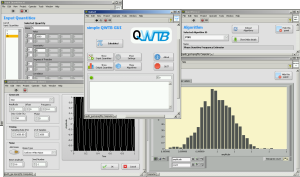# QWTB

Software Toolbox for Sampling Measurements

#QWTB is a software toolbox written in M-code and is running in MATLAB or GNU Octave. It aims for aggregation of high-quality algorithms required for data processing of sampled measurements. QWTB consist of data processing algorithms from different sources, unifying application interface and graphical user interface.

The toolbox gives the possibility to use different data processing algorithms with one set of data and removes the need to reformat data for every particular algorithm. Toolbox is extensible. The toolbox can variate input data and calculate uncertainties by means of Monte Carlo Method (MCM).

Toolbox does not require to modify source code of Algorithms, it usually adapts.

Typical use case: you make a sampling and you want to: get frequency spectra, calculate amplitude and phase of main sine signal and calculate Allan deviation. But you do not want to search algorithms, to learn how to use these algorithms and to reformat variables for every one algorithm. Solution? QWTB!

## QWTB can help with:

• searching of useful algorithms,
• learning how to use algorithms,
• application of algorithms to a user data.

## QWTB has:

• standardized format of (input/output) quantities independent on the required algorithm,
• standardized use of algorithms,
• examples for every implemented algorithm,
• full documentation with examples,
• integration into LabVIEW - QWTBLVLib
• graphical user interface - simple QWTB GUI
• ability to variate inputs and calculate uncertainties by means of Monte Carlo Method.
• tests for every implemented algorithm.

## QWTB is:

• easy to use,
• open source,
• extensible.

QWTB is hosted on GitHub. You can either download the latest release, development version, or take a look at GitHub webpage, see links on the top of this page.

## Documentation

Documentation is accessible on GitHub, or use direct links (developement version):
QWTB documentation - Toolbox description.
QWTB documentation - Implemented algorithms.

## LabVIEW library

A LabVIEW library QWTBLVLib was developed to easily integrate QWTB into user projects. For now, the library requires working copy of MATLAB. In the future a link to the GNU Octave is planned.

## Graphical User Interface

A simple QWTB GUI was developed in LABView. It is aimed as an example of QWTBLVLib implementation. How to install and how to use is shortly presented in following video.## How to cite:

QWTB will be presented on Conference on Precision Electromagnetic Measurements, 10-15 July, 2016